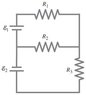# Determine the magnitudes and directions of the currents in each R's.

• NasuSama

## Homework Statement

Determine the magnitudes and directions of the currents in each resistor shown in the figure. The batteries have emfs of ##\varepsilon_1 = 9.1## V and ##\varepsilon_2 = 11.6## V and the resistors have values of ##R_1 = 15 \Omega## , ##R_2 = 33 \Omega## , and ##R_3 = 30 \Omega## .

Assume each battery has internal resistance ##1.3 \Omega##.

## Homework Equations

EMF equation: ##\varepsilon = IR##

## The Attempt at a Solution

The equations for this case are:

##\varepsilon_1 = I_1(R_1 + r) + I_2(R_2 + r)##
##\varepsilon_2 = I_3(R_3 + r) + I_2(R_2 + r)##

where ##r## is the internal resistance. I know that by junction rule, ##I_2 = I_1 + I_3##

I found that ##I_1 \approx 0.092## and ##I_3 \approx 0.13##. For ##I_2##, the value is approximately 0.22 in terms of two significant figures, but Mastering Physics marked it incorrect, indicating that I need to check my calculations. I don't know what I did wrong. 0.23 and 0.21 are also incorrect.

#### Attachments

•26n34.jpg
5.1 KB · Views: 931
The thing here is that I still get the same calculations over and over again, but Mastering Physics marked it wrong for no reason. Will anyone check the calculation? Thank you!

Why would you add the internal resistance of the battery to R2? R2 is not in series with either battery. Otherwise, your equations look okay. Fix the battery resistance issue and I think you'll be okay.